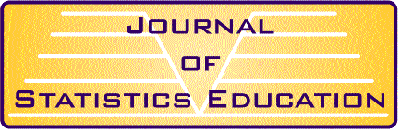Subscription Information 2000 Contents and Abstracts 1999 Contents and Abstracts JSE Archive (1993-1998) Index to Articles JSE Information Service Data Archive JSE Editorial Board Information for JSE Authors Information for Data Contributors ASA Publications2000 Abstracts

### Volume 8, Number 3 (November 2000)

#### Scott Preston, "Teaching Prediction Intervals" (214K)

Teaching prediction intervals to introductory audiences presents unique opportunities. In this article I present a strategy for involving students in the development of a nonparametric prediction interval. Properties of the resulting procedure, as well as related concepts and similar procedures that appear throughout statistics, may be illustrated and investigated within the concrete context of the data. I suggest a generalization of the usual normal theory prediction interval. This generalization, in tandem with the nonparametric method, results in an approach to prediction that may be systematically deployed throughout a course in introductory statistics.

Key Words: Confidence interval; Descriptive statistic; Error reduction; Normal distribution; Percentile.

#### K. L. Weldon, "A Simplified Introduction to Correlation and Regression" (34K)

The simplest forms of regression and correlation involve formulas that are incomprehensible to many beginning students. The application of these techniques is also often misunderstood. The simplest and most useful description of the techniques involves the use of standardized variables, the root mean square operation, and certain distance measures between points and lines. On the standardized scale, the simple linear regression coefficient equals the correlation coefficient, and the distinction between fitting a line to points and choosing a line for prediction is made transparent. The typical size of prediction errors is estimated in a natural way by summarizing the actual prediction errors incurred in the dataset by use of the regression line for prediction. The connection between correlation and distance is simplified. Despite their intuitive appeal, few textbooks make use of these simplifications in introducing correlation and regression.

Key Words: Distance; Prediction error; Root mean square; Standard deviation; Standardized variables.

#### Annette F. Gourgey, "A Classroom Simulation Based on Political Polling To Help Students Understand Sampling Distributions" (35K)

Sampling distributions are central to understanding statistical inference, yet they are one of the most difficult concepts for introductory statistics students. Although hands-on teaching methods are preferred, finding the right balance between theory and practical experience has not been easy. Simulation activities have not always captured the research situations that statisticians work with. This paper describes a method developed by the author to teach sampling distributions using a collaborative learning simulation based on political polling. Anecdotally, students found the polling scenario easy to understand, interesting, and enjoyable, and they were able to explain the meaning of sample results and inferences about the population. Sample examination questions are included, with examples of students' responses that suggest that the method helped them to understand sampling error and its role in statistical inference.

Key Words: Collaborative learning; Introductory statistics; Math anxiety; Standard error; Statistical inference.

#### Vicki Stover Hertzberg, W. Scott Clark, and Donna J. Brogan, "Developing Pedagogical and Communications Skills in Graduate Students: The Emory University Biostatistics TATTO Program" (23K)

Key Words: Biostatistical consulting; Statistical pedagogy; Teacher training.

#### Alan McLean, "The Predictive Approach to Teaching Statistics" (50K)

Statistics is commonly taught as a set of techniques to aid in decision making, by extracting information from data. It is argued here that the underlying purpose, often implicit rather than explicit, of every statistical analysis is to establish one or more probability models that can be used to predict values of one or more variables. Such a model constitutes 'information' only in the sense, and to the extent, that it provides predictions of sufficient quality to be useful for decision making. The quality of the decision making is determined by the quality of the predictions, and hence by that of the models used.

Using natural criteria, the 'best predictions' for nominal and numeric variables are, respectively, the mode and mean. For a nominal variable, the quality of a prediction is measured by the probability of error. For a numeric variable, it is specified using a prediction interval. Presenting statistical analysis in this way provides students with a clearer understanding of what a statistical analysis is, and its role in decision making.

Key Words: Prediction; Prediction interval; Probability model.

#### "Teaching Bits: A Resource for Teachers of Statistics" (36K)

This column features "bits" of information sampled from a variety of sources that may be of interest to teachers of statistics. Bob delMas abstracts information from the literature on teaching and learning statistics, while Bill Peterson summarizes articles from the news and other media that may be used with students to provoke discussions or serve as a basis for classroom activities or student projects.

Editorial Board for Volume 8, Number 3

Subscription Information | Current Issue | JSE Archive (1993-1998) | Data Archive | Index | Search JSE | JSE Information Service | Editorial Board | Information for Authors | Contact JSE | ASA Publications